Modern Algebra: An Introduction - 6 Edition - Chapter 9 - Problem 9.15
Register Now

Join StudySoup

Get Full Access to Modern Algebra: An Introduction - 6 Edition - Chapter 9 - Problem 9.159780470384435

# For x, Y E lR, let X ~ Y mean that X - Y is an integer. Verify that "'- is an

Modern Algebra: An Introduction | 6th Edition

Problem 9.15

For x, Y E lR, let X ~ Y mean that X - Y is an integer. Verify that "'- is an equivalence relation.Describe the equivalence classes geometrically, with the elements of JR identified with thepoints on a line in the usual way. Give a complete set of equivalence class representatives.

Accepted Solution
Step-by-Step Solution:

Step 1 of 3

For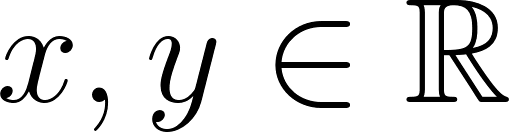, let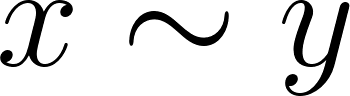mean that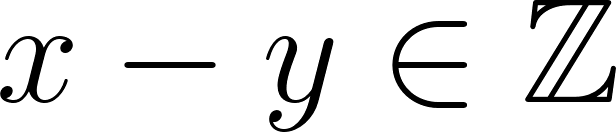Since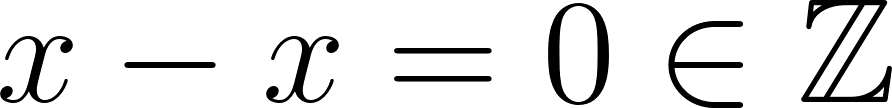for all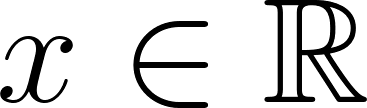we have thatfor alland therefore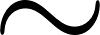is reflexive.

###### Chapter 9, Problem 9.15 is Solved

Step 2 of 3

Step 3 of 3

Unlock Textbook Solution